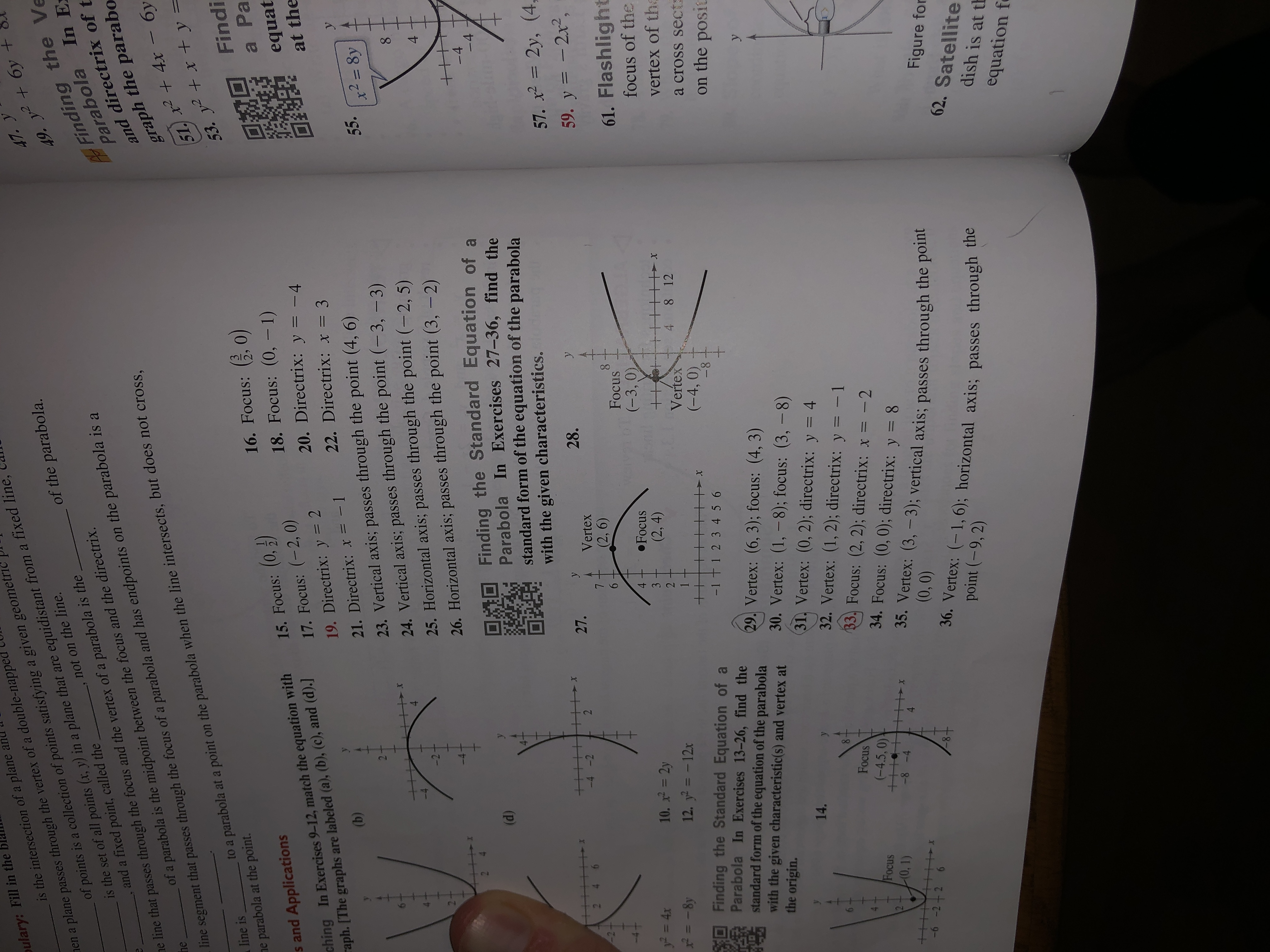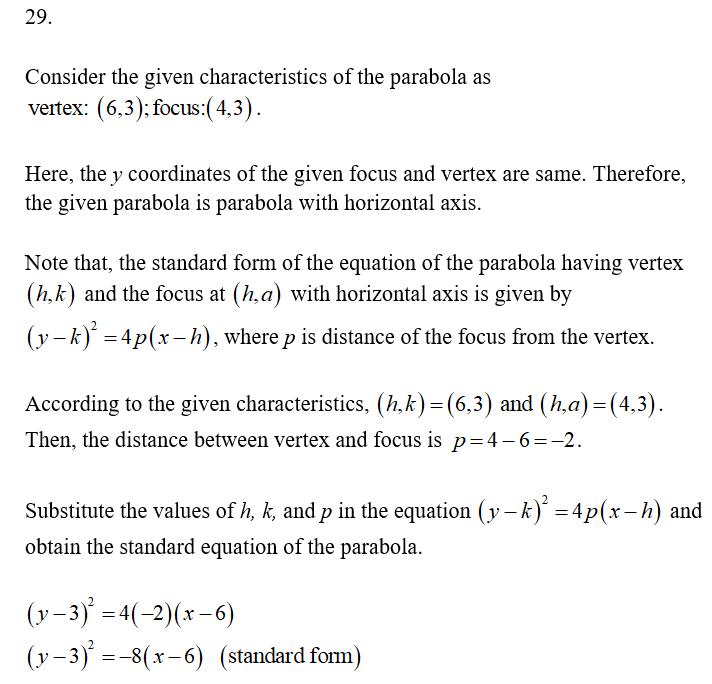# oulary: Fill in theIs the set of all points (r, y) in a plane that are equidistant from a fixed line,and a fixed point, called theis the intersection of a plane annen a plane passes through the vertex of a double-napped47. yof points is a collection of points satisfying a given geometricof the parabola.Finding the VeParabola In E:and directrix of tgraph the parabo51. x2 +4x – 6y53. y2+x + y =Findinot on the line.49. y² + 6y +ne line that passes through the focus and the vertex of a parabola is theheof a parabola is the midpoint between the focus and the directrix.ine segment that passes through the focus of a parabola and has endpoints on the parabola is aline isto a parabola at a point on the parabola when the line intersects, but does not crossne parabola at the point.15. Focus: (0,)17. Focus: (-2, 0)16. Focus: , 0)18. Focus: (0, –1)20. Directrix: y = -4s and Applicationsching In Exercises 9-12, match the equation withraph. [The graphs are labeled (a), (b), (c), and (d).]a Paequat%3D19. Directrix: y = 222. Directrix: x = 3at the(b)21. Directrix: x = -123. Vertical axis; passes through the point (4, 6)24. Vertical axis; passes through the point (-3, –3)25. Horizontal axis; passes through the point (-2, 5)26. Horizontal axis; passes through the point (3, -2)55.x2 = 8y%3D4.-28.10 Finding the Standard Equation of aParabola In Exercises 27-36, find thestandard form of the equation of the parabola(d)-4-4with the given characteristics.++++57. x = 2y, (4,%3D21-4 -227.28.Vertex(2,6)59. y = - 2x2,8.Focusy = 4x61. Flashlight410. x = 2y•Focus(-3, 0)|3D3= -8y12. y = - 12x(2, 4)focus of the2%3DVertex8 12vertex of thaFinding the Standard Equation of aParabola In Exercises 13-26, find thestandard form of the equation of the parabolawith the given characteristic(s) and vertex atthe origin.(-4, 0)-8-1 t1 2 3 4 5 6a cross sects6.on the posia29. Vertex: (6, 3); focus: (4, 3)30. Vertex: (1, –8); focus: (3, –8)31. Vertex: (0, 2); directrix: y = 414.8-Focus(-4.5, 0)11++-8 -432. Vertex: (1, 2); directrix: y = - 133. Focus: (2, 2); directrix: x = -2Focus10,1)34. Focus: (0, 0); directrix: y = 835. Vertex: (3, -3); vertical axis; passes through the pome(0,0)4-6 -2+ 2 636. Vertex: (-1, 6); horizontal axis; passes through thepoint (-9, 2)Figure for62. Satellitedish is at thequation f4-2.++++

Question
12 views

How do you answer 29 and 31?

(This is not graded but, rather, an exercise to achieve acquisition).help_outlineImage Transcriptioncloseoulary: Fill in the Is the set of all points (r, y) in a plane that are equidistant from a fixed line, and a fixed point, called the is the intersection of a plane an nen a plane passes through the vertex of a double-napped 47. y of points is a collection of points satisfying a given geometric of the parabola. Finding the Ve Parabola In E: and directrix of t graph the parabo 51. x2 +4x – 6y 53. y2+x + y = Findi not on the line. 49. y² + 6y + ne line that passes through the focus and the vertex of a parabola is the he of a parabola is the midpoint between the focus and the directrix. ine segment that passes through the focus of a parabola and has endpoints on the parabola is a line is to a parabola at a point on the parabola when the line intersects, but does not cross ne parabola at the point. 15. Focus: (0,) 17. Focus: (-2, 0) 16. Focus: , 0) 18. Focus: (0, –1) 20. Directrix: y = -4 s and Applications ching In Exercises 9-12, match the equation with raph. [The graphs are labeled (a), (b), (c), and (d).] a Pa equat %3D 19. Directrix: y = 2 22. Directrix: x = 3 at the (b) 21. Directrix: x = -1 23. Vertical axis; passes through the point (4, 6) 24. Vertical axis; passes through the point (-3, –3) 25. Horizontal axis; passes through the point (-2, 5) 26. Horizontal axis; passes through the point (3, -2) 55. x2 = 8y %3D 4. -2 8. 10 Finding the Standard Equation of a Parabola In Exercises 27-36, find the standard form of the equation of the parabola (d) -4 -4 with the given characteristics. ++ ++ 57. x = 2y, (4, %3D 21 -4 -2 27. 28. Vertex (2,6) 59. y = - 2x2, 8. Focus y = 4x 61. Flashlight 4 10. x = 2y •Focus (-3, 0) |3D 3 = -8y 12. y = - 12x (2, 4) focus of the 2 %3D Vertex 8 12 vertex of tha Finding the Standard Equation of a Parabola In Exercises 13-26, find the standard form of the equation of the parabola with the given characteristic(s) and vertex at the origin. (-4, 0) -8 -1 t1 2 3 4 5 6 a cross sects 6. on the posia 29. Vertex: (6, 3); focus: (4, 3) 30. Vertex: (1, –8); focus: (3, –8) 31. Vertex: (0, 2); directrix: y = 4 14. 8- Focus (-4.5, 0) 11++ -8 -4 32. Vertex: (1, 2); directrix: y = - 1 33. Focus: (2, 2); directrix: x = -2 Focus 10,1) 34. Focus: (0, 0); directrix: y = 8 35. Vertex: (3, -3); vertical axis; passes through the pome (0,0) 4 -6 -2+ 2 6 36. Vertex: (-1, 6); horizontal axis; passes through the point (-9, 2) Figure for 62. Satellite dish is at th equation f 4- 2. ++++ fullscreen
check_circle

Step 1...

### Want to see the full answer?

See Solution

#### Want to see this answer and more?

Solutions are written by subject experts who are available 24/7. Questions are typically answered within 1 hour.*

See Solution
*Response times may vary by subject and question.
Tagged in